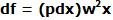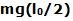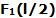## Tuesday, March 10, 2009

### Irodov Problem 1.281As the rod AB spins with angular velocity w, it experiences a centrifugal force f pulling it outwards (in the reference frame spinning with the rod). Let us first compute the value of the force f.

Consider an infinitesimally small piece of the rod AB of length dx at a distance x from the axis OO'. Let the linear density (mass per unit length) of the rod be p. Then the mass of this infinitesimally small piece of the rod is pdx. As this piece spins at a rate w, it experiences a centrifugal forceThe total centrifugal force experienced by the rod AB can be found by integrating over the entire the rod as,Let the horizontal components of the forces acting at the pivots at the ends of the rod O' and O be forces F1 and F2. Since the (rod + axis) system does not have any acceleration in the horizontal direction we have,Now let us consider all the torques acting on the (axis+rod) system about an axis passing through point A perpendicular to the plane of OO' and AB. There are three torques i) due to the gravitational force mg acting at the center of the rod AB given byin the clockwise direction, ii) the torque due to the force F2 equal toacting in the clockwise direction and iii) the torque due to force F1 equal toacting in the anti-clockwise direction. Since the (rod+axis) system is not experiencing any angular acceleration we have,From (1), (2) and (3) we have,Setting F2=0 we have,and